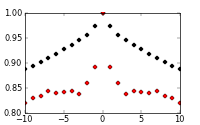# autocorrelation question

Friends,
I want to plot the autocorrelation of my data as a function of time as given in the following link, with autocorrelation coef in Y axis and Time in x-axis.

http://www.itl.nist.gov/div898/handbook/eda/section3/autocopl.htm

I used the following code to achieve the same using the acorr function. But the acorr function doesnt seem to plot the autocorrelation coef. as a function of time. Why is it so ?. The output figure is attached. I want to plot the time dependent decay of my values.

import matplotlib.pyplot as plt
import numpy as np

#Assignments
FIG=plt.figure(figsize=(4.0,2.5),dpi=50)
NR=1;NC=1
cols=[‘black’,‘red’]
ax1.xcorr(data1,data1,color=cols,usevlines=False)
ax1.acorr(data2,color=cols,usevlines=False)
plt.savefig(‘auto.png’,dpi=50)
plt.show()···

C. Balasubramanian

Hi bala,

what is the input? How did you calculate it?

Francesco

2012/6/19 Bala subramanian <bala.biophysics@…287…>

···

Friends,
I want to plot the autocorrelation of my data as a function of time as given in the following link, with autocorrelation coef in Y axis and Time in x-axis.

http://www.itl.nist.gov/div898/handbook/eda/section3/autocopl.htm

I used the following code to achieve the same using the acorr function. But the acorr function doesnt seem to plot the autocorrelation coef. as a function of time. Why is it so ?. The output figure is attached. I want to plot the time dependent decay of my values.

import matplotlib.pyplot as plt
import numpy as np

#Assignments
FIG=plt.figure(figsize=(4.0,2.5),dpi=50)

NR=1;NC=1
cols=[‘black’,‘red’]
ax1.xcorr(data1,data1,color=cols,usevlines=False)
ax1.acorr(data2,color=cols,usevlines=False)

plt.savefig(‘auto.png’,dpi=50)
plt.show()

C. Balasubramanian

Live Security Virtual Conference

Exclusive live event will cover all the ways today’s security and

threat landscape has changed and how IT managers can respond. Discussions

will include endpoint security, mobile security and the latest in malware

Matplotlib-users mailing list

Matplotlib-users@lists.sourceforge.net

https://lists.sourceforge.net/lists/listinfo/matplotlib-users

Cordiali saluti, Dr.Oteri Francesco

Hi,
The input is a parameter termed dipole moment. I want to see how it decays with respect to time.

···

On Tue, Jun 19, 2012 at 11:12 AM, francesco oteri <francesco.oteri@…287…> wrote:

Hi bala,
what is the input? How did you calculate it?

Francesco

2012/6/19 Bala subramanian <bala.biophysics@…287…>

Friends,
I want to plot the autocorrelation of my data as a function of time as given in the following link, with autocorrelation coef in Y axis and Time in x-axis.

http://www.itl.nist.gov/div898/handbook/eda/section3/autocopl.htm

I used the following code to achieve the same using the acorr function. But the acorr function doesnt seem to plot the autocorrelation coef. as a function of time. Why is it so ?. The output figure is attached. I want to plot the time dependent decay of my values.

import matplotlib.pyplot as plt
import numpy as np

#Assignments
FIG=plt.figure(figsize=(4.0,2.5),dpi=50)

NR=1;NC=1
cols=[‘black’,‘red’]
ax1.xcorr(data1,data1,color=cols,usevlines=False)
ax1.acorr(data2,color=cols,usevlines=False)

plt.savefig(‘auto.png’,dpi=50)
plt.show()

C. Balasubramanian

Live Security Virtual Conference

Exclusive live event will cover all the ways today’s security and

threat landscape has changed and how IT managers can respond. Discussions

will include endpoint security, mobile security and the latest in malware

Matplotlib-users mailing list

Matplotlib-users@lists.sourceforge.net

https://lists.sourceforge.net/lists/listinfo/matplotlib-users

Cordiali saluti, Dr.Oteri Francesco

C. Balasubramanian